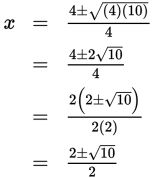# SAT Math Multiple Choice Question 934: Answer and Explanation

### Test Information

Question: 934

2. For what values of x is the equation 2x2 = 4x + 3 true?

• A.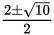• B.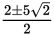• C.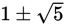• D.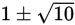Explanation:

A

Difficulty: Medium

Getting to the Answer: First, write the equation in the form ax2 + bx + c = 0: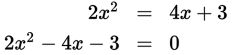Next, jot down the values that you'll need: a = 2, b = -4, and c = -3. Then, substitute these values into the quadratic formula and simplify: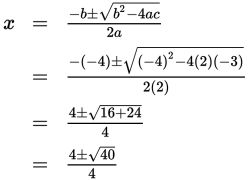This isn't one of the answer choices, so you'll need to simplify the radical: